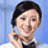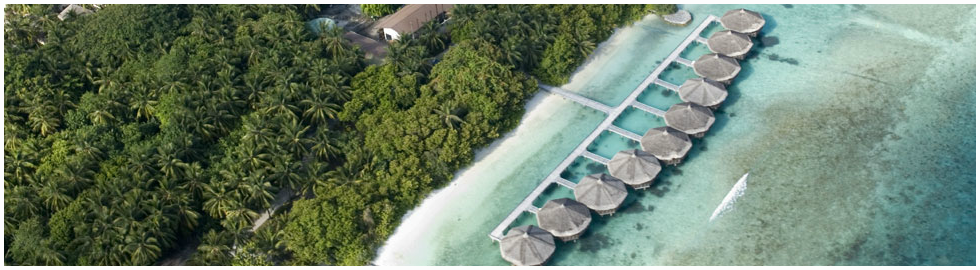\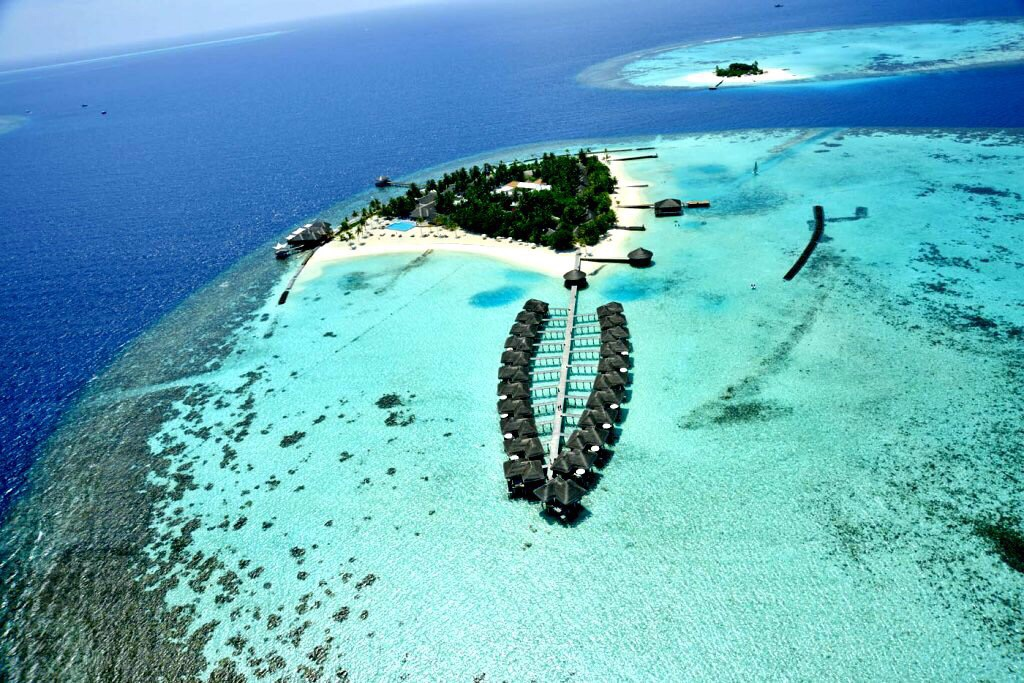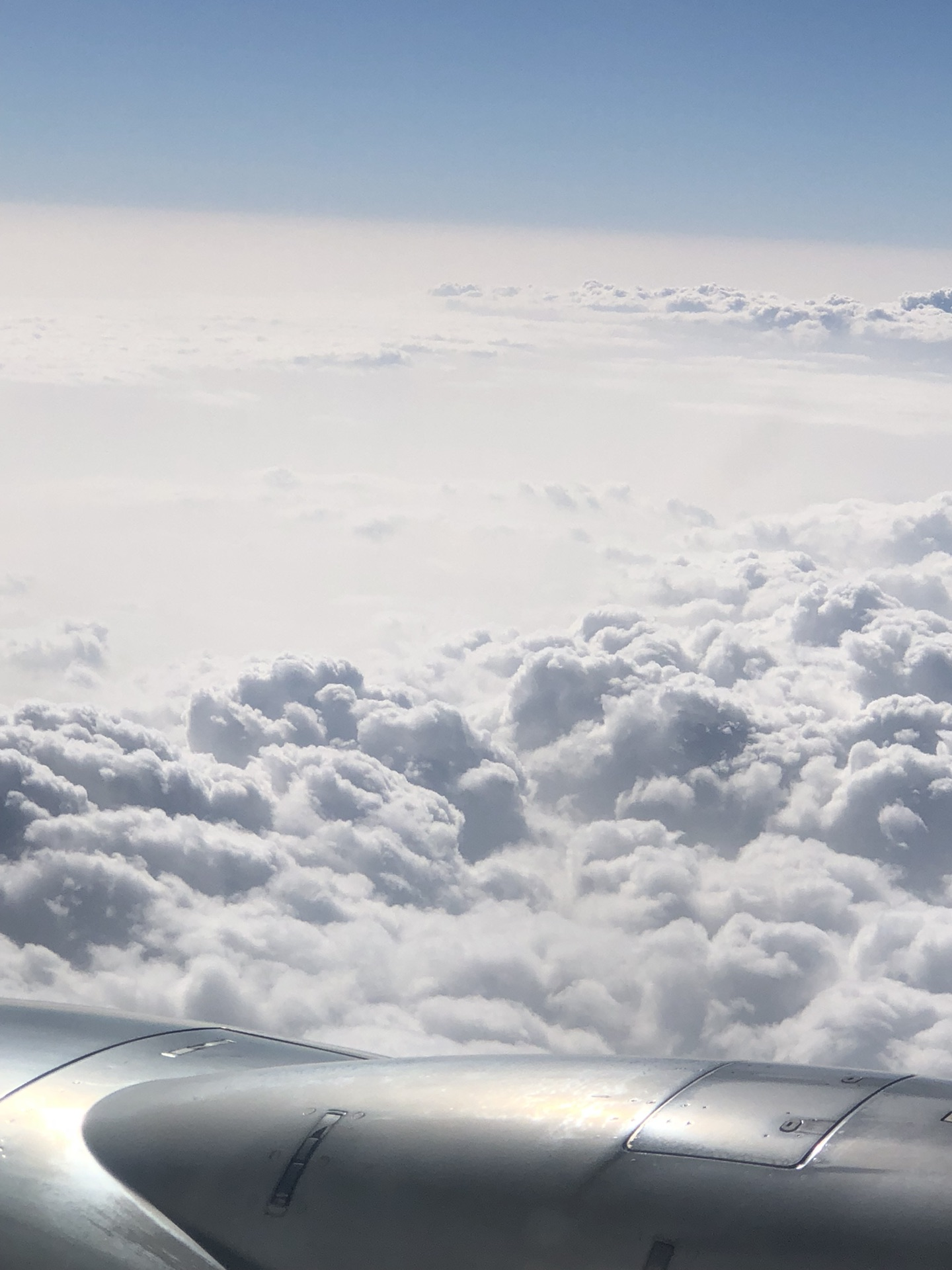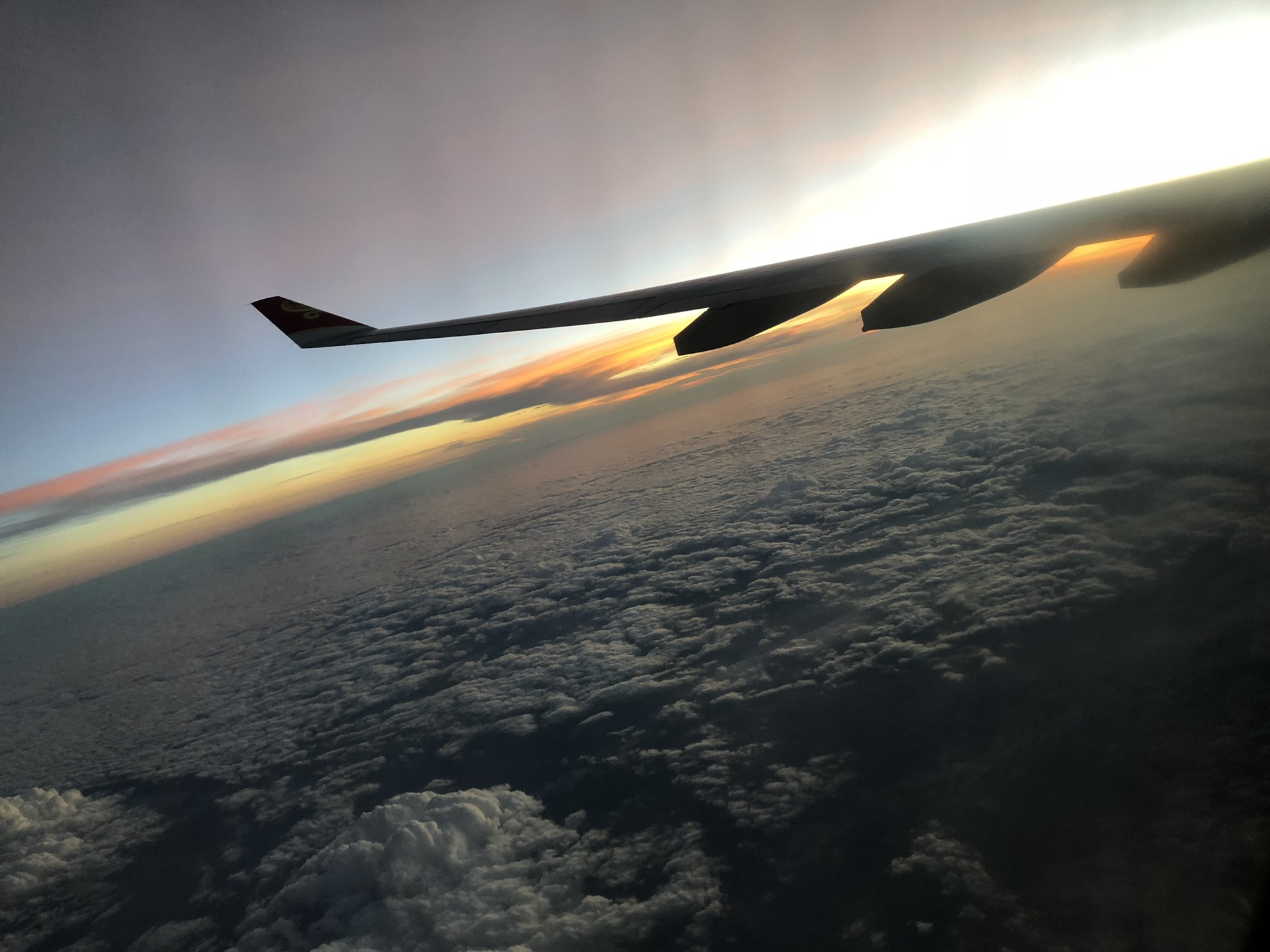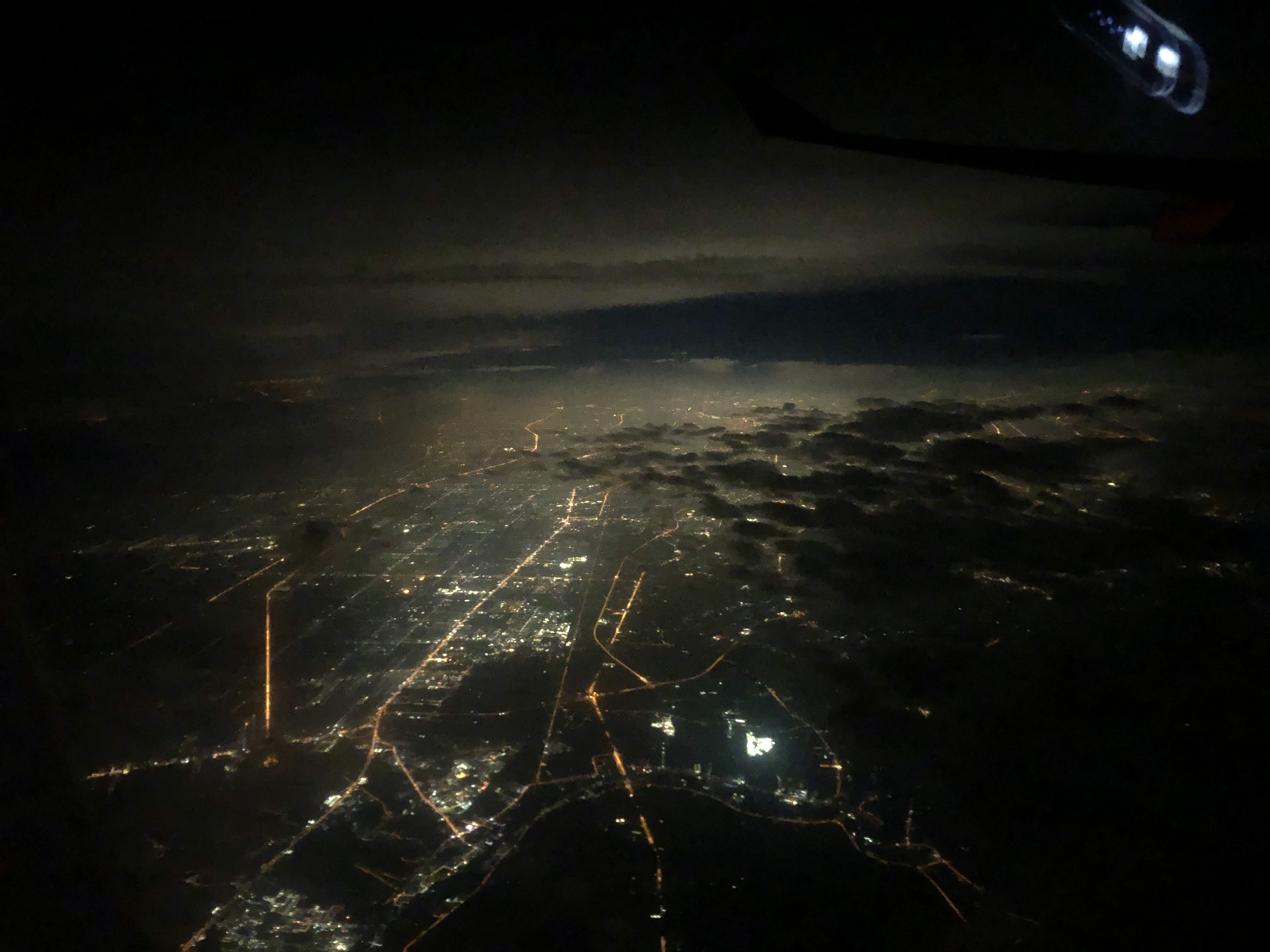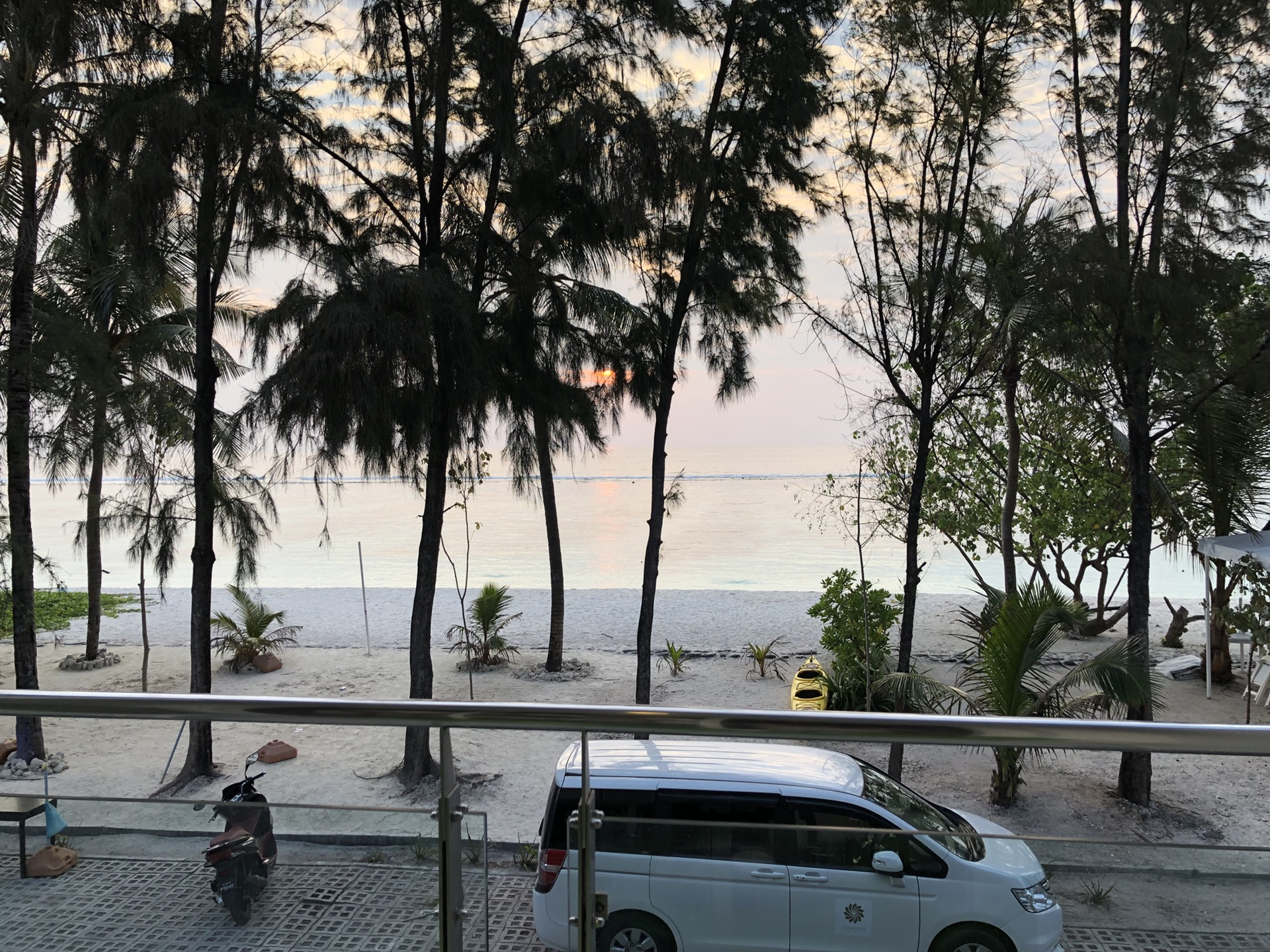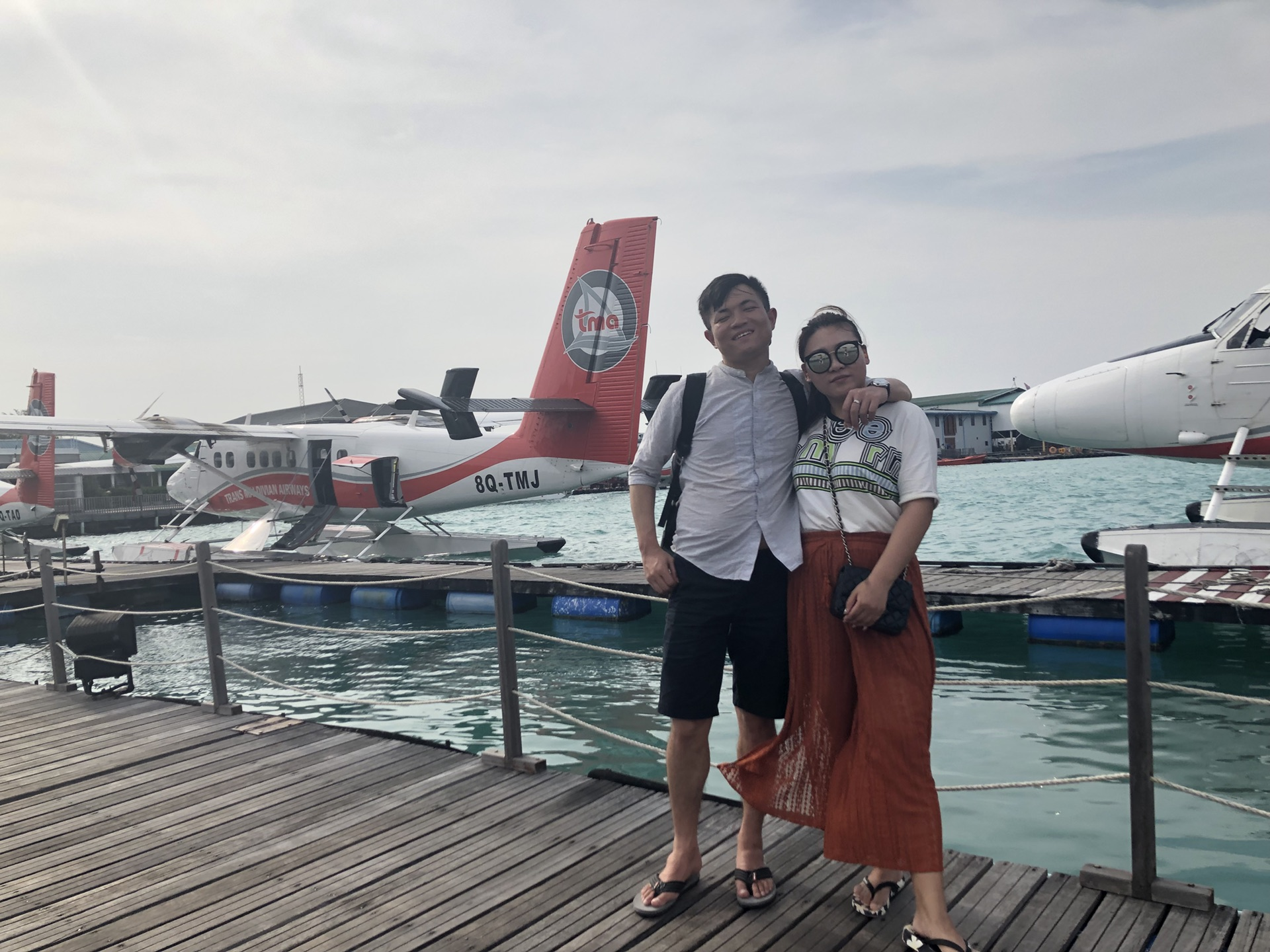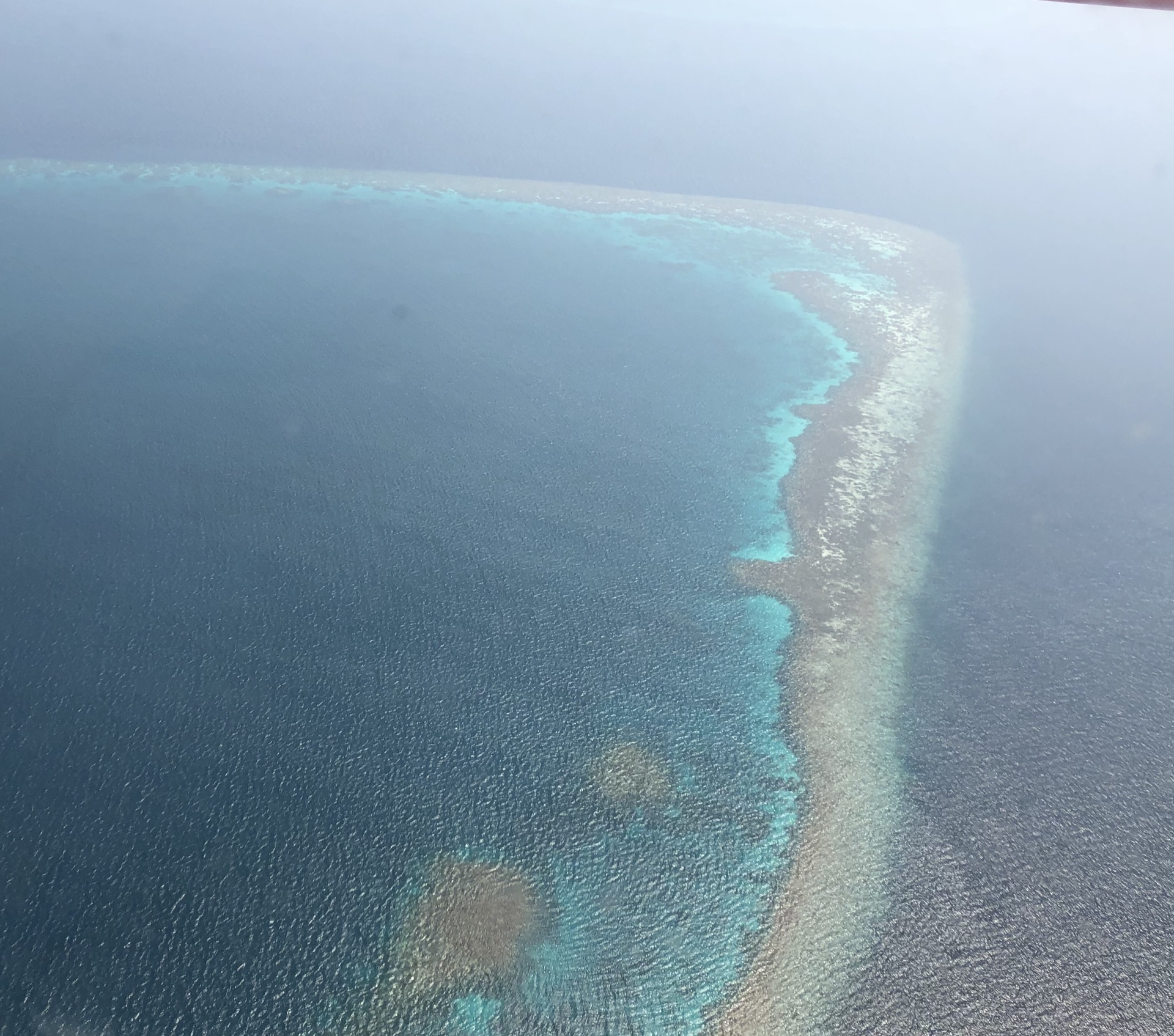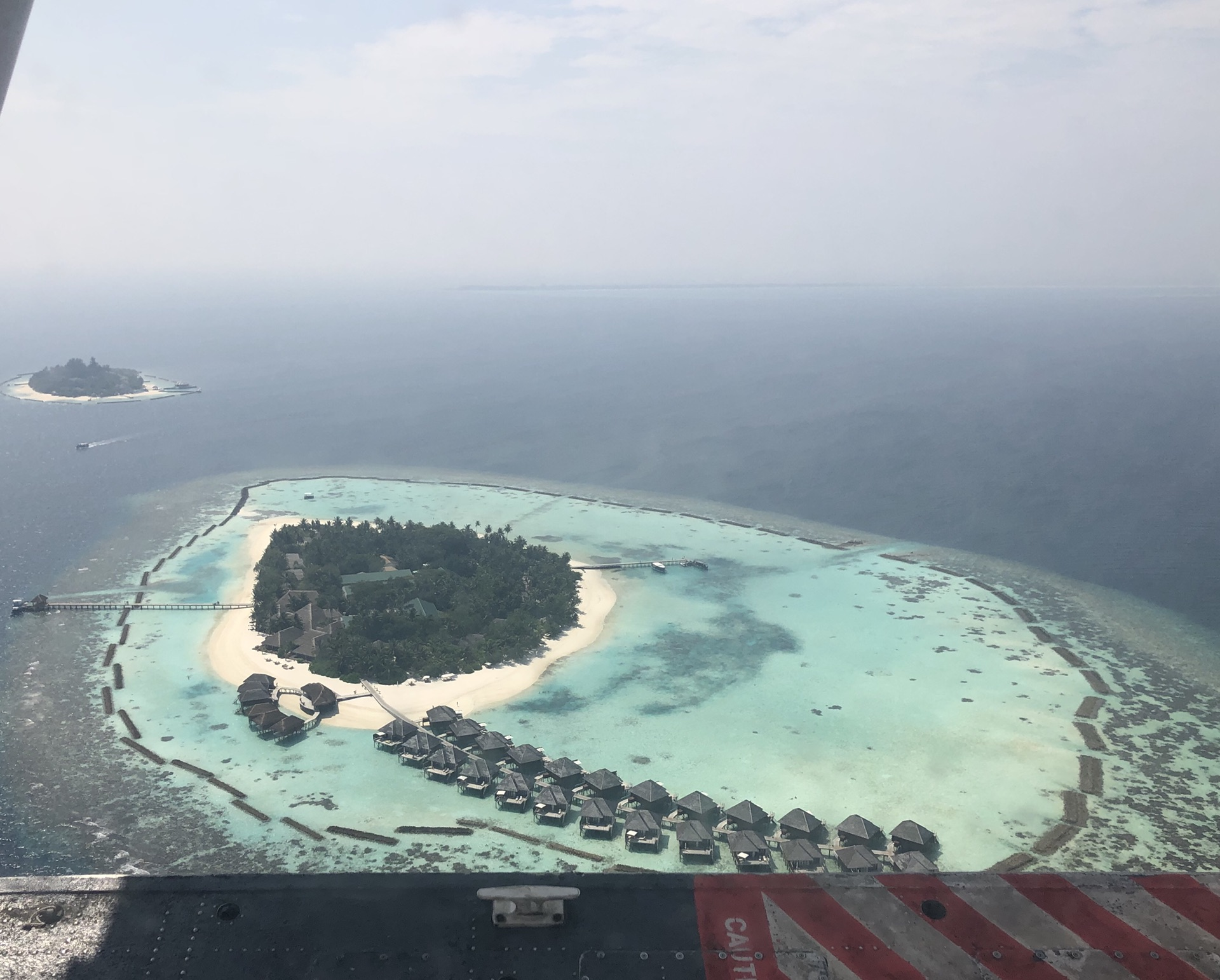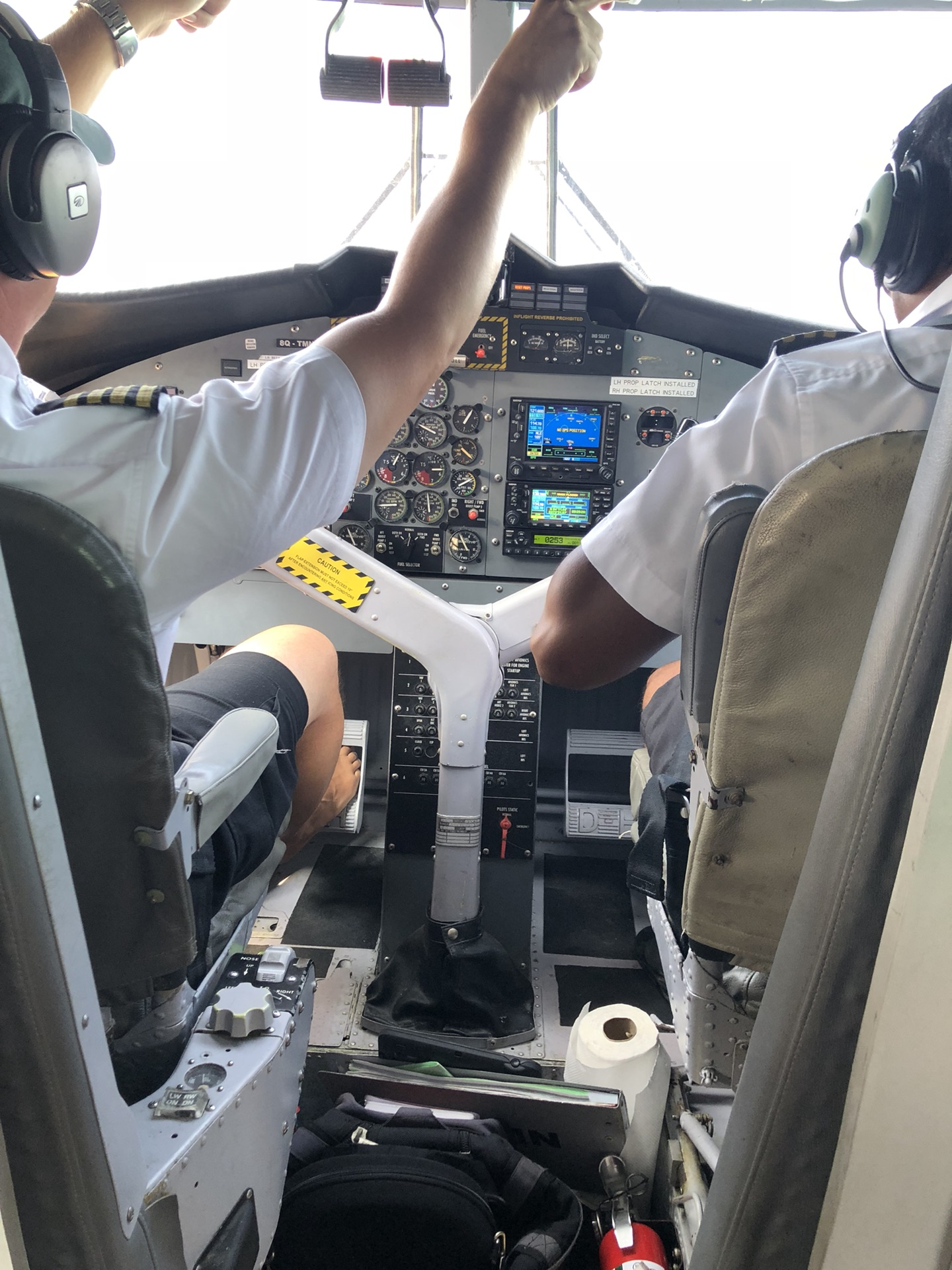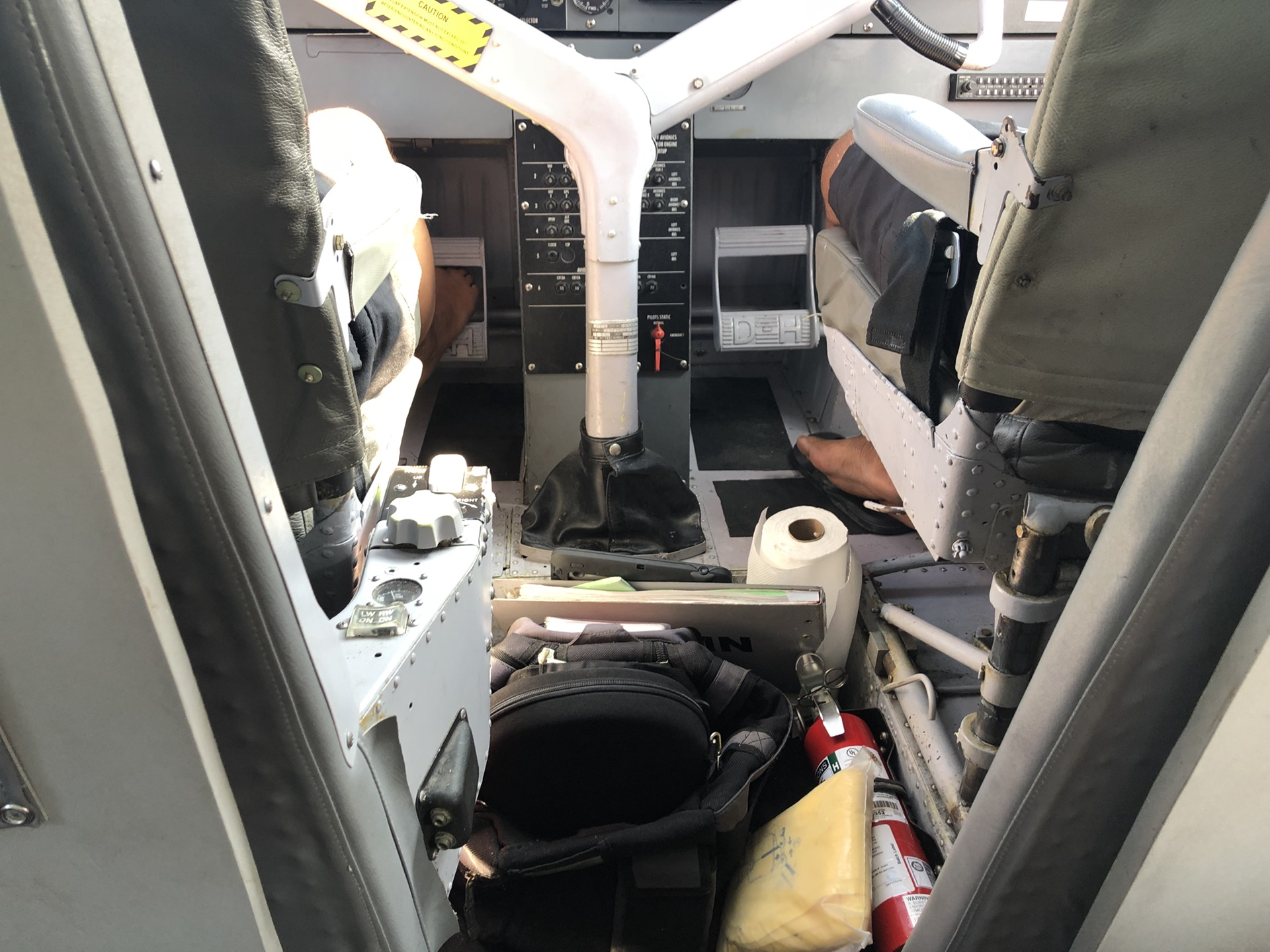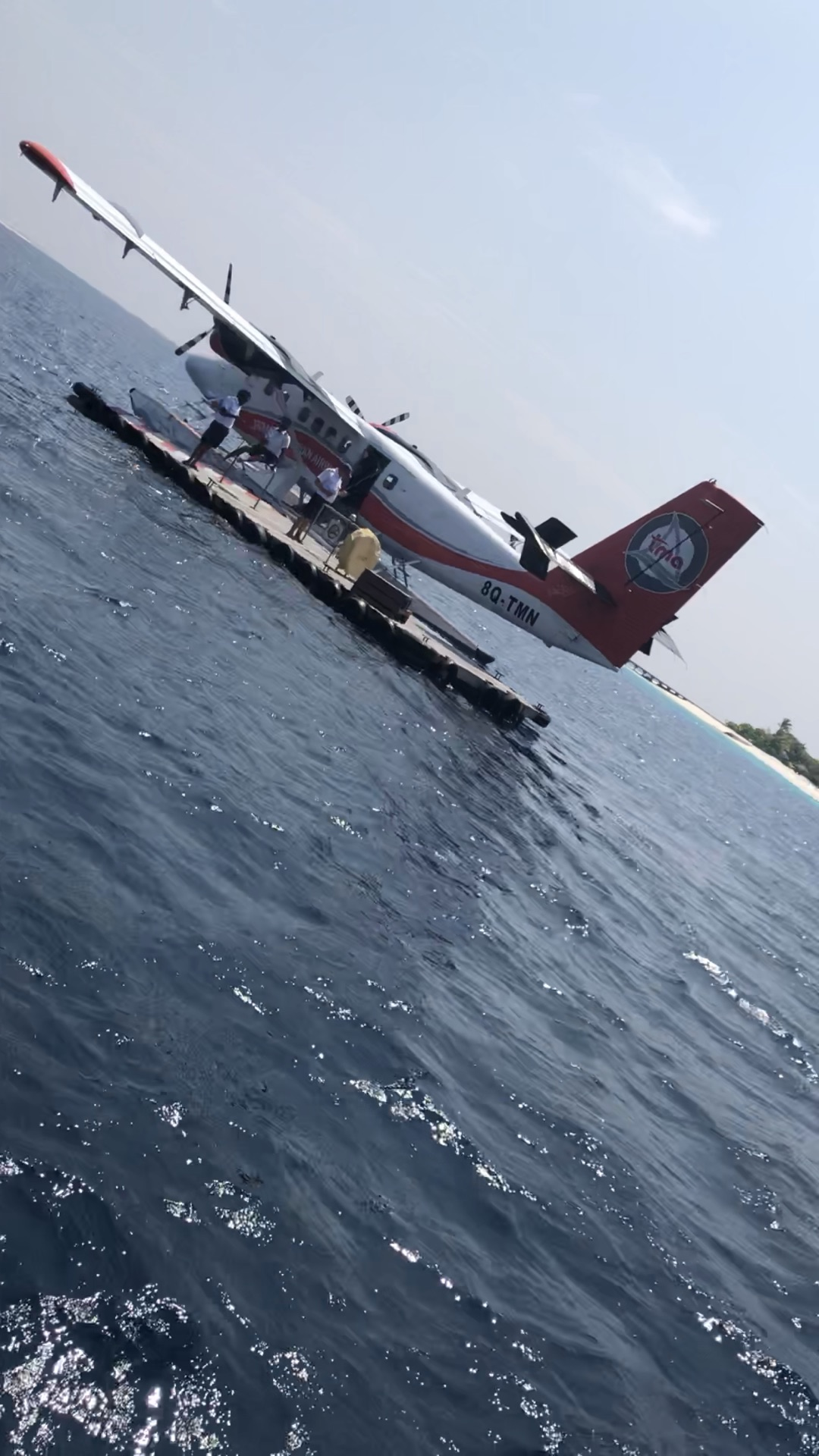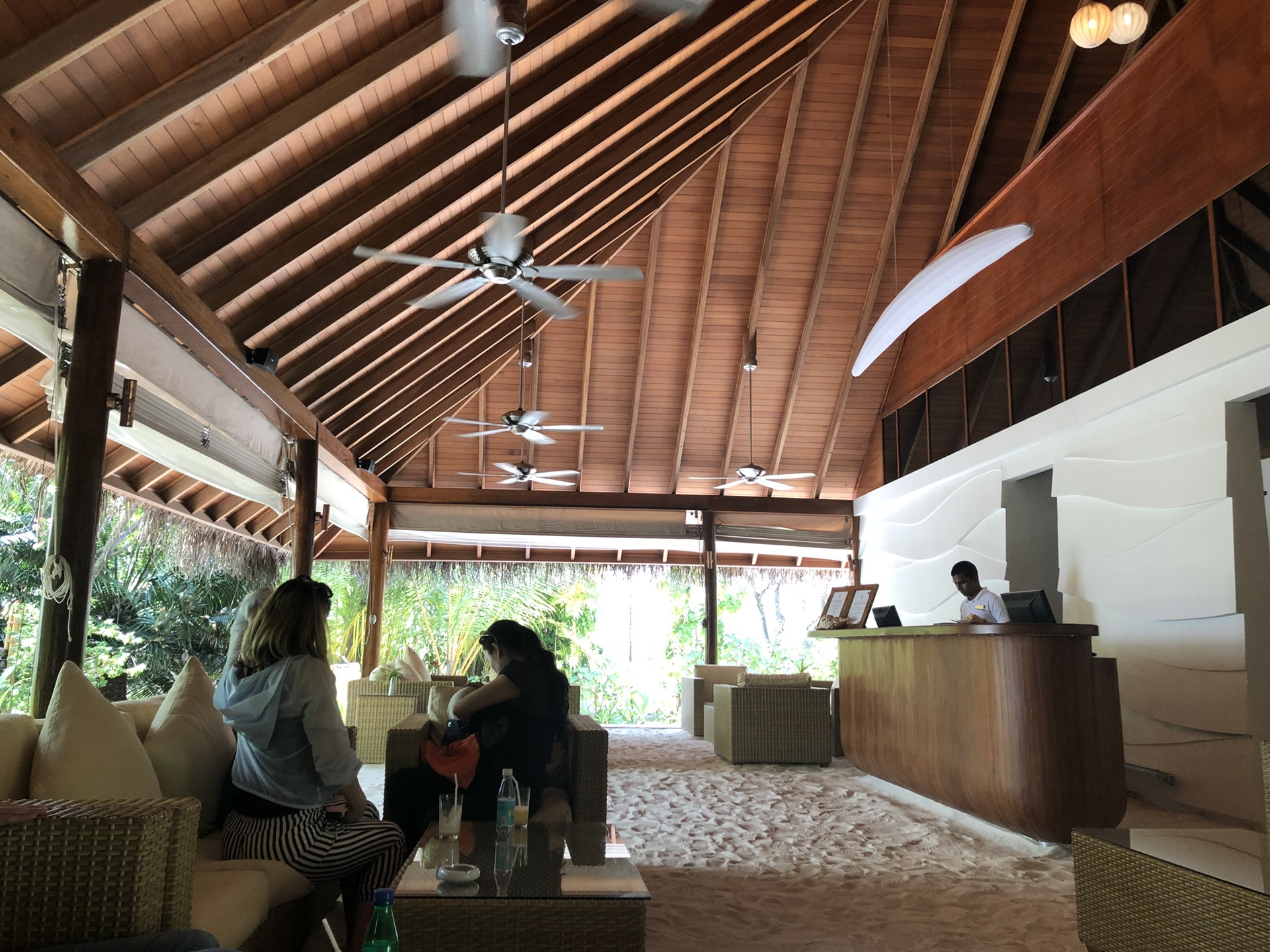<div id="_j_photo_300494207" class="add_pic _j_anchorcnt _j_seqitem" style="margin:" 0px="" 18px;="" padding:="" 0px;="" word-wrap:="" break-word;="" position:="" relative;="" color:="" rgb(68,="" 68,="" 68);="" font-family:="" arial,="" "lucida="" grande",="" "microsoft="" yahei",="" "hiragino="" sans="" gb",="" gb="" w3",="" simsun,="" "pingfang="" sc",="" stheiti;="" font-size:="" 15px;="" white-space:="" normal;="" width:="" 680px;="">•• 迷失之旅—马尔代夫马富士瓦鲁maafushivaru
• 期待下一次的马代 我的心迷失在哪一望无际的深海中的国度 马尔代夫——马富士瓦鲁 padding:=""0px;...

Power by 海岸线假期 & 海岸线国旅